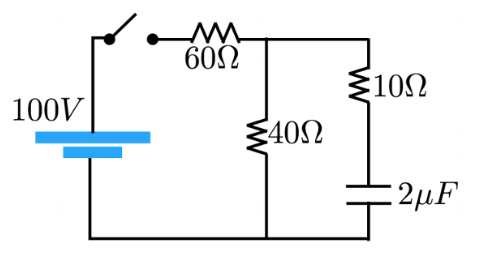# Problem: RC Circuit Analysis After the switch is closed, does the current through the resistor increase, decrease or stay the same?

###### FREE Expert Solution

The charging current for a capacitor:

$\overline{){\mathbf{I}}{\mathbf{=}}\frac{{\mathbf{V}}_{\mathbf{b}\mathbf{a}\mathbf{t}\mathbf{t}\mathbf{e}\mathbf{r}\mathbf{y}}}{\mathbf{R}}{{\mathbf{e}}}^{\mathbf{-}\mathbf{\left(}\mathbf{t}\mathbf{/}\mathbf{R}\mathbf{C}\mathbf{\right)}}}$

The current decreases exponentially toward zero. That is, e-∞ = 0.

92% (274 ratings)###### Problem Details

RC Circuit Analysis

After the switch is closed, does the current through the resistor increase, decrease or stay the same?Frequently Asked Questions

What scientific concept do you need to know in order to solve this problem?

Our tutors have indicated that to solve this problem you will need to apply the !! Resistor-Capacitor Circuits concept. If you need more !! Resistor-Capacitor Circuits practice, you can also practice !! Resistor-Capacitor Circuits practice problems.